# Selina Solutions Concise Maths Class 10 Chapter 8 Remainder and Factor Theorems

In order to enhance the performance of students in ICSE Class 10 exam, we at BYJU’S have designed solutions for Mathematics under Selina Solutions for Class 10 Mathematics. Subject experts have created the solutions according to the requirements of all the students. Remainder theorem, factor theorem and their uses are the key concepts covered in this chapter. For further assistance, students can get Selina Solutions for Class 10 Mathematics Chapter 8 Remainder and Factor theorems PDF, from the links provided below.

## Selina Solutions Concise Maths Class 10 Chapter 8 Remainder and Factor Theorems Download PDF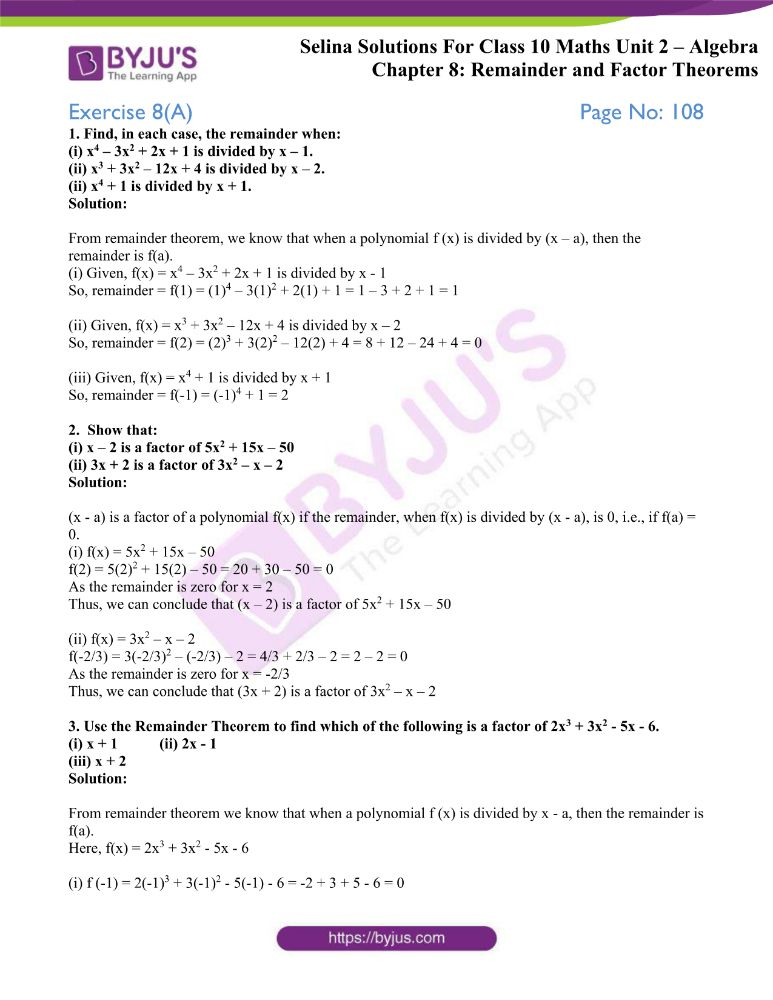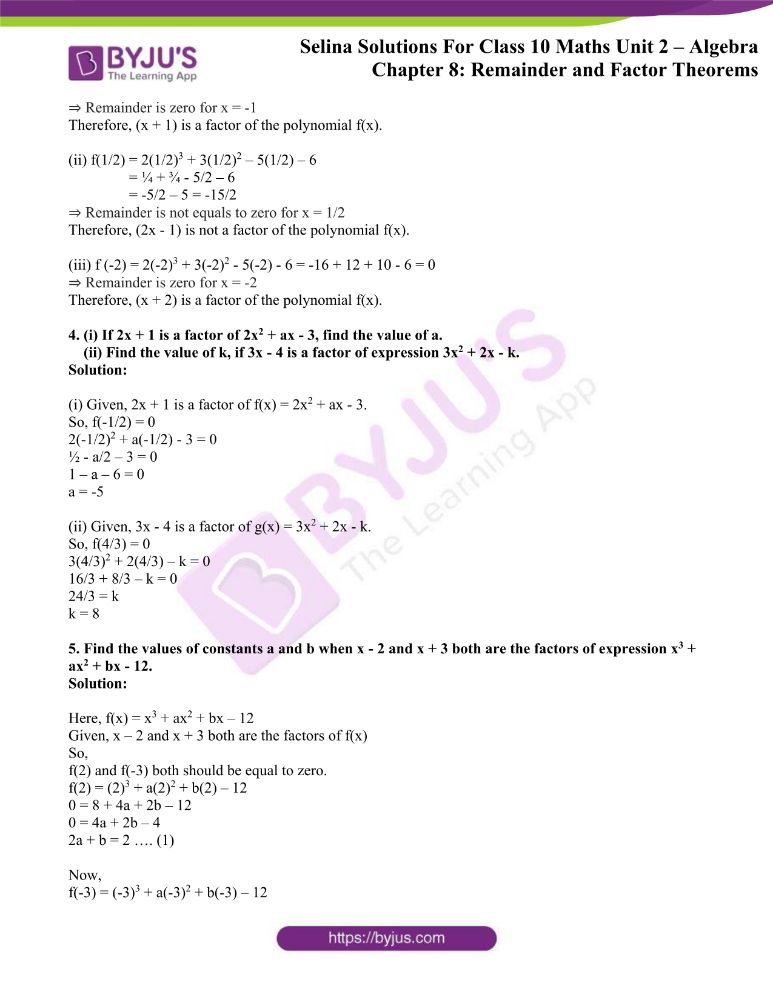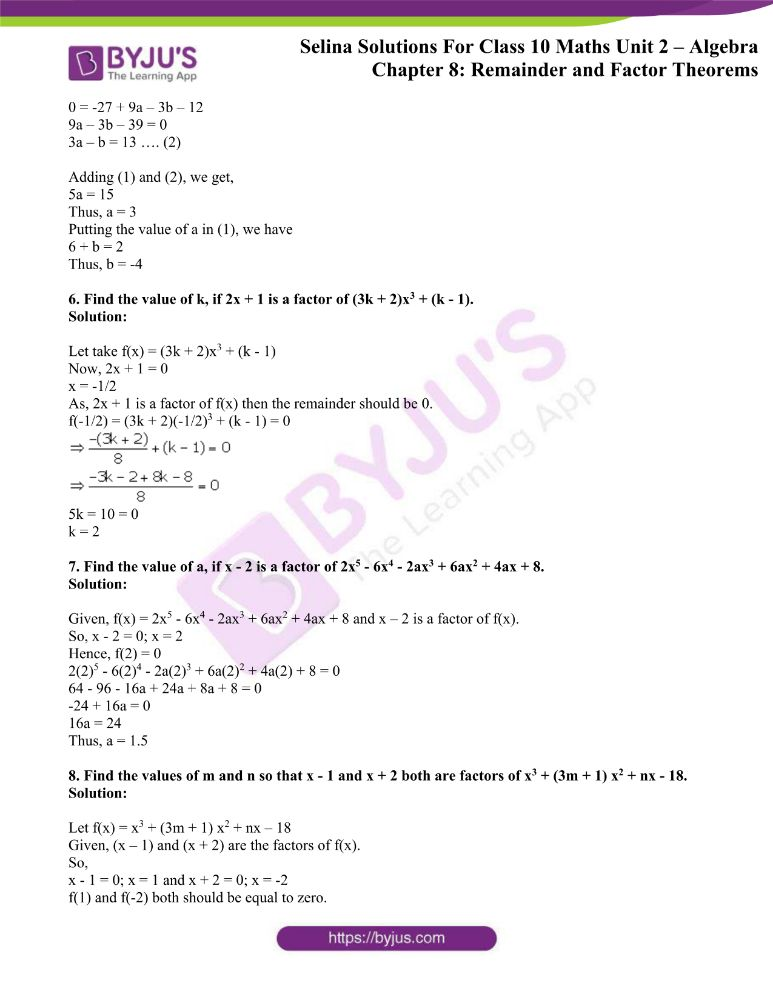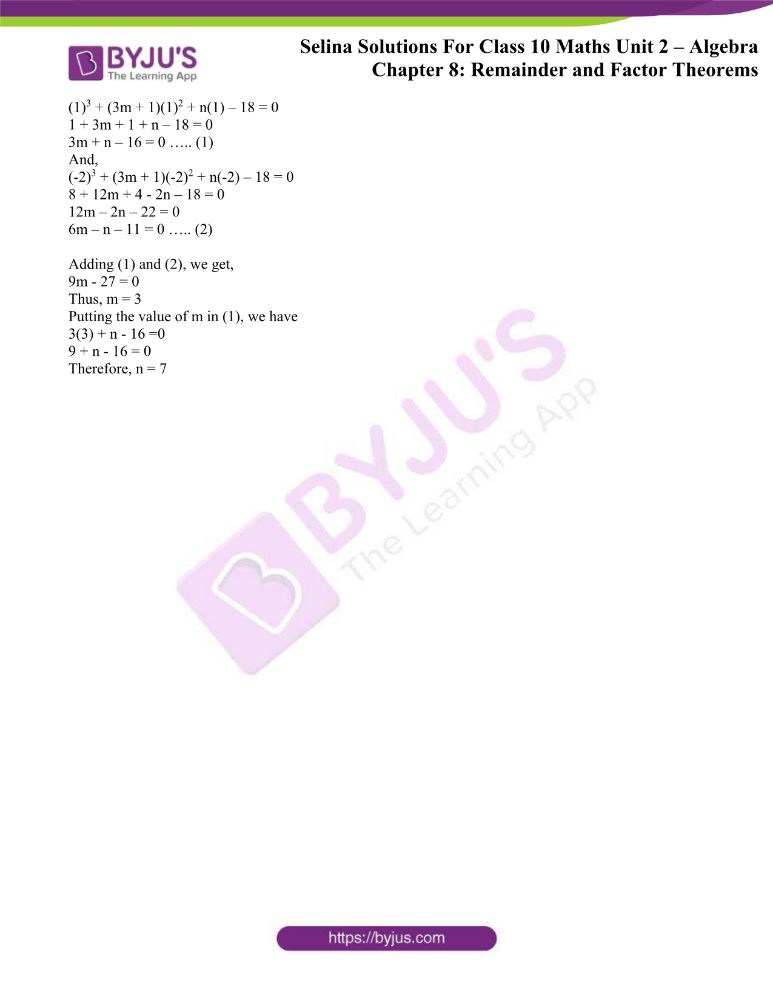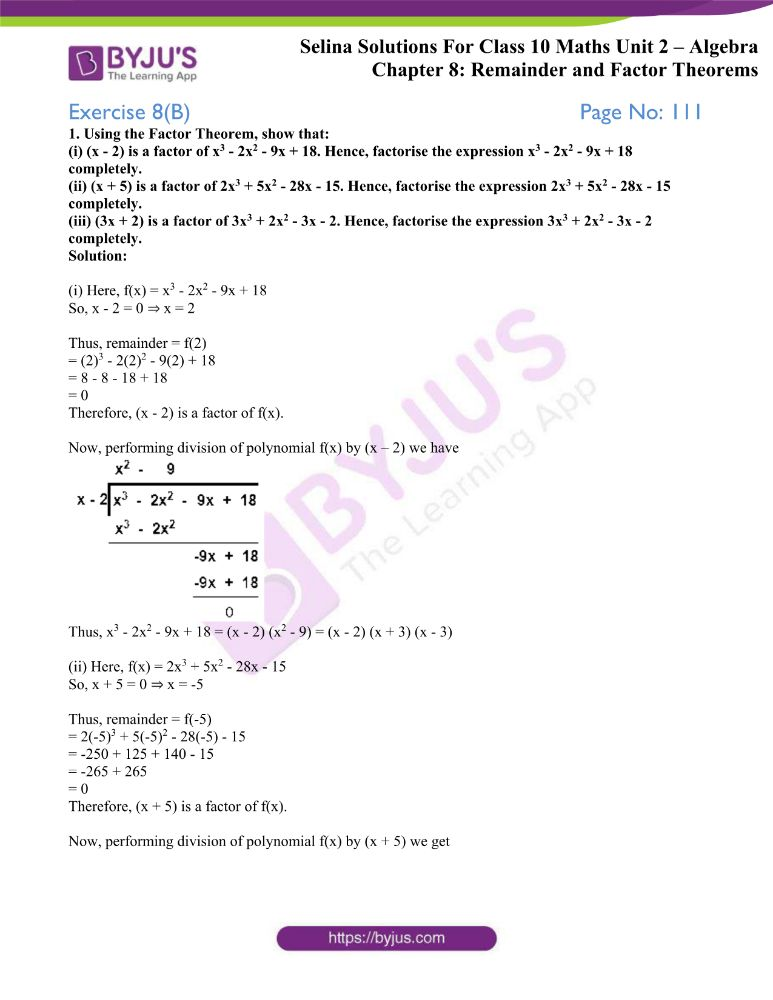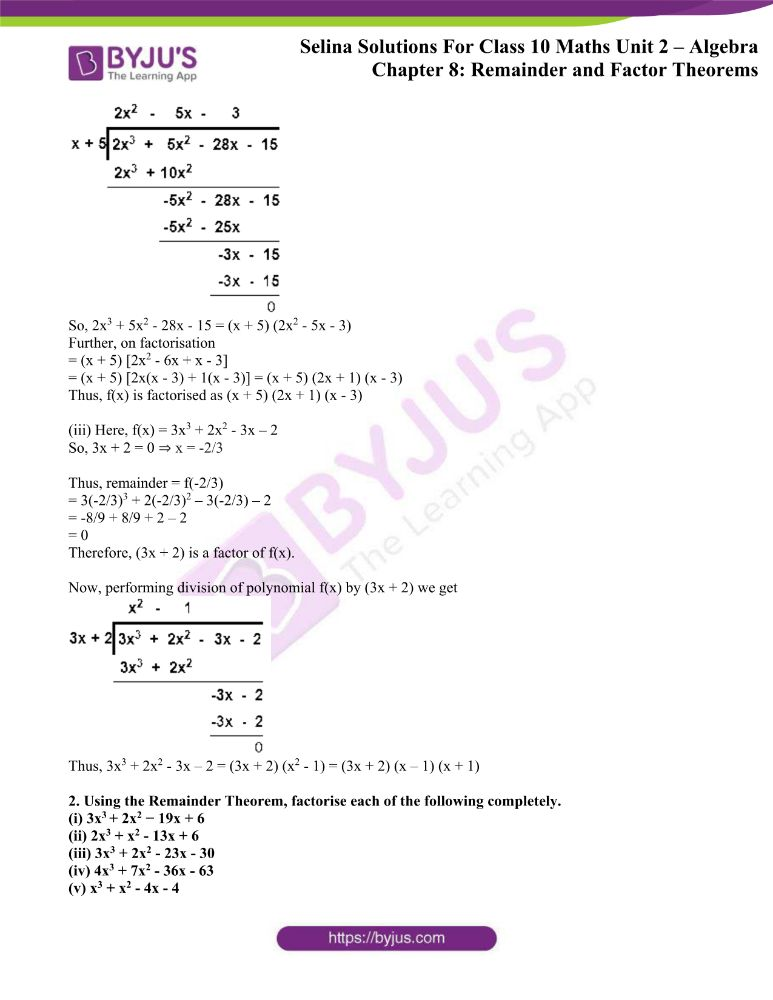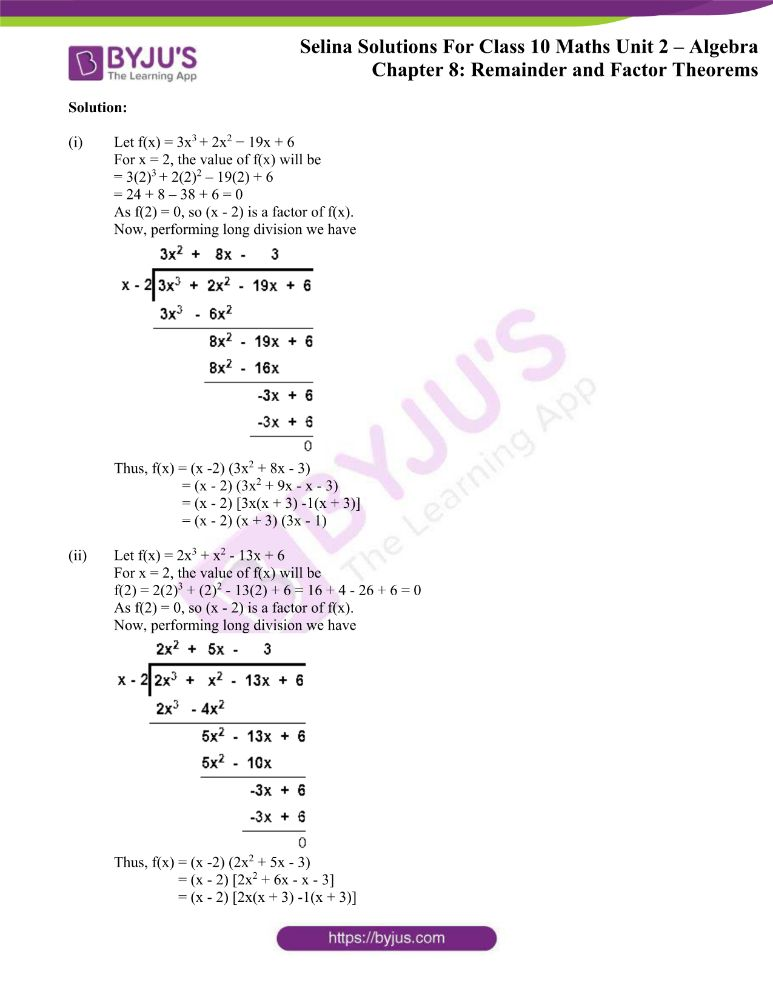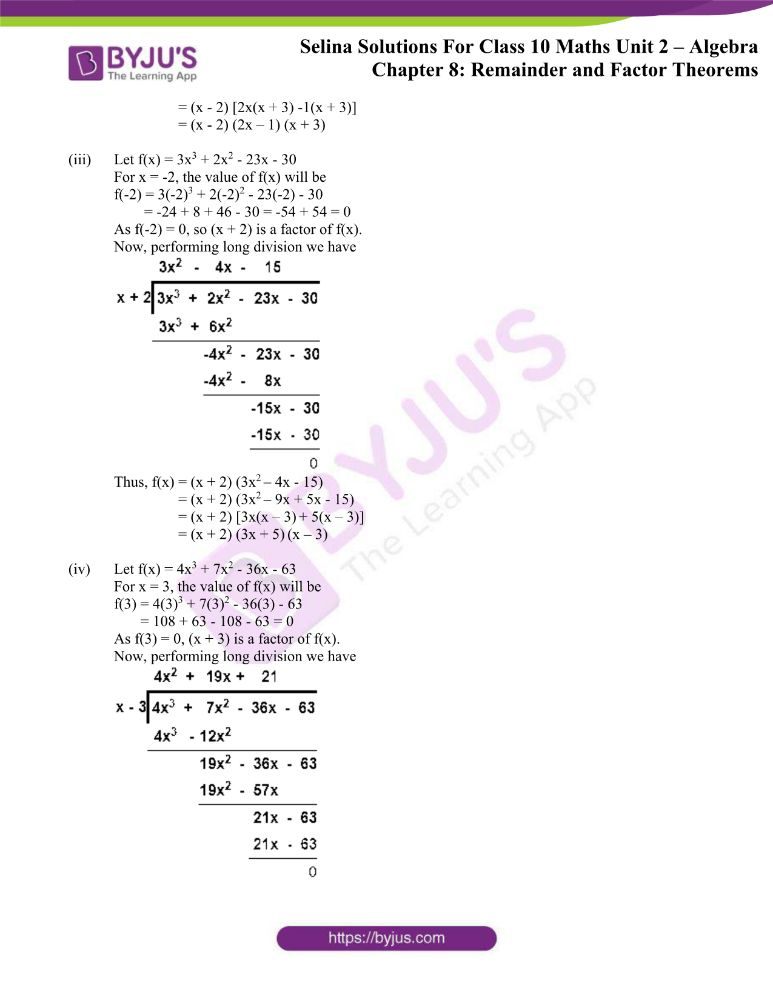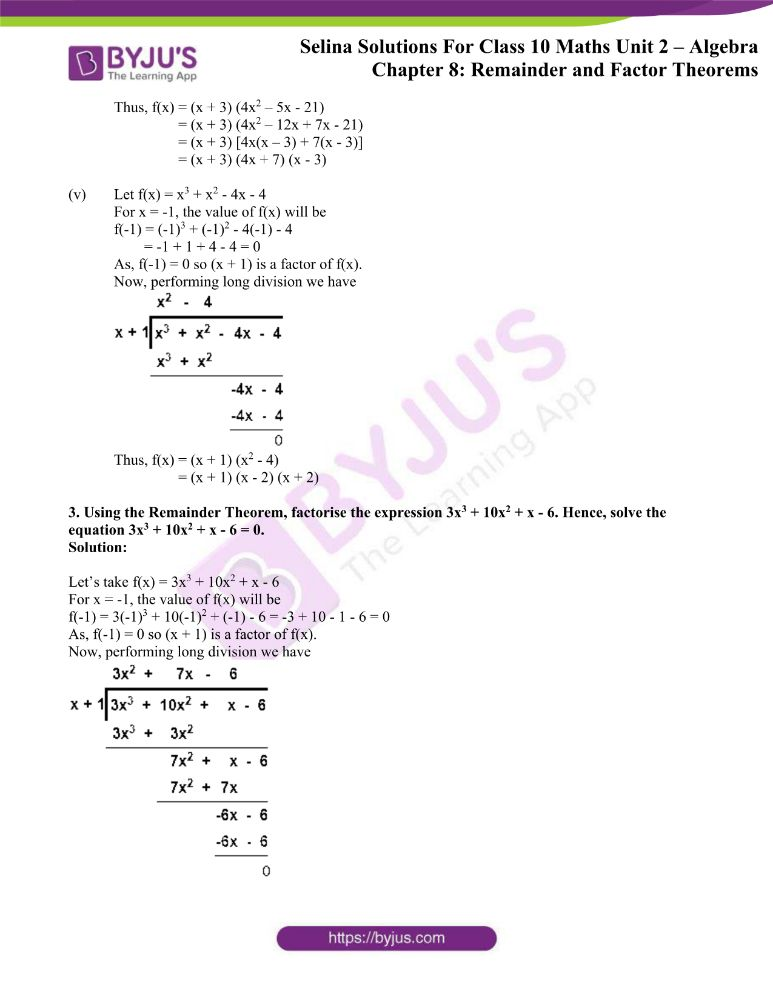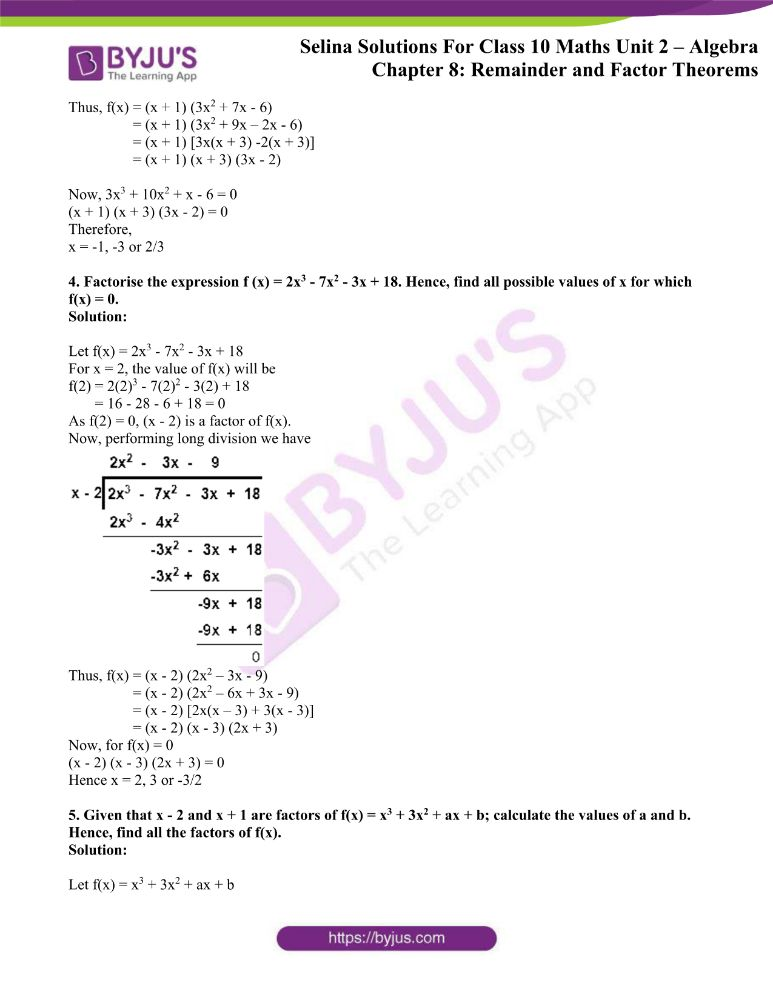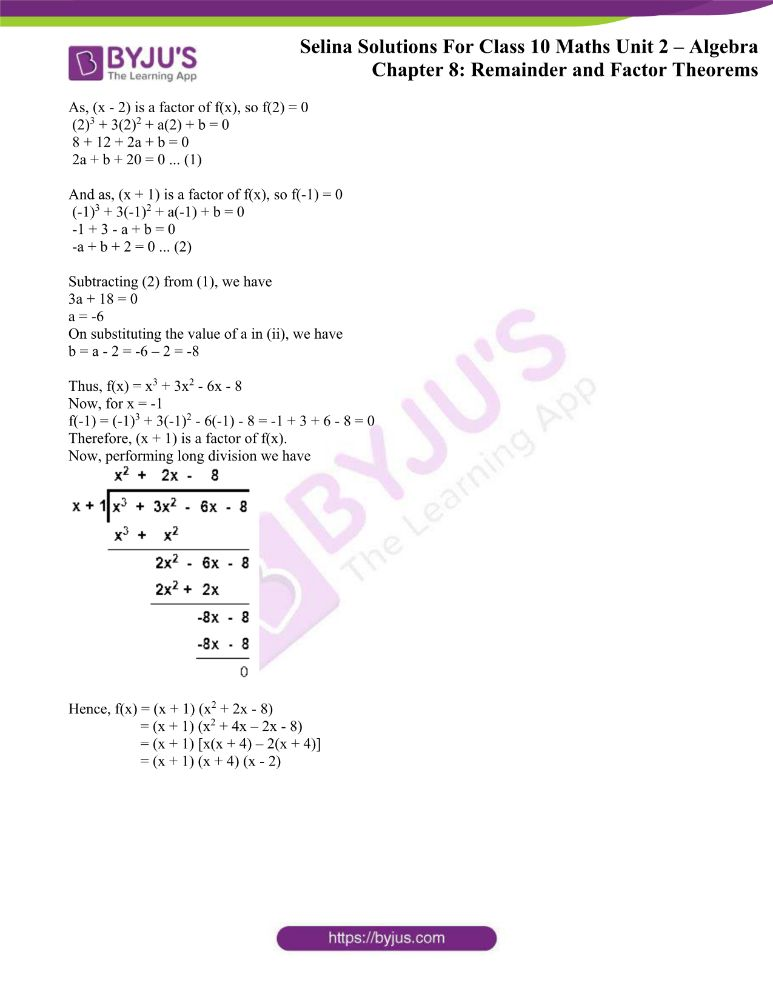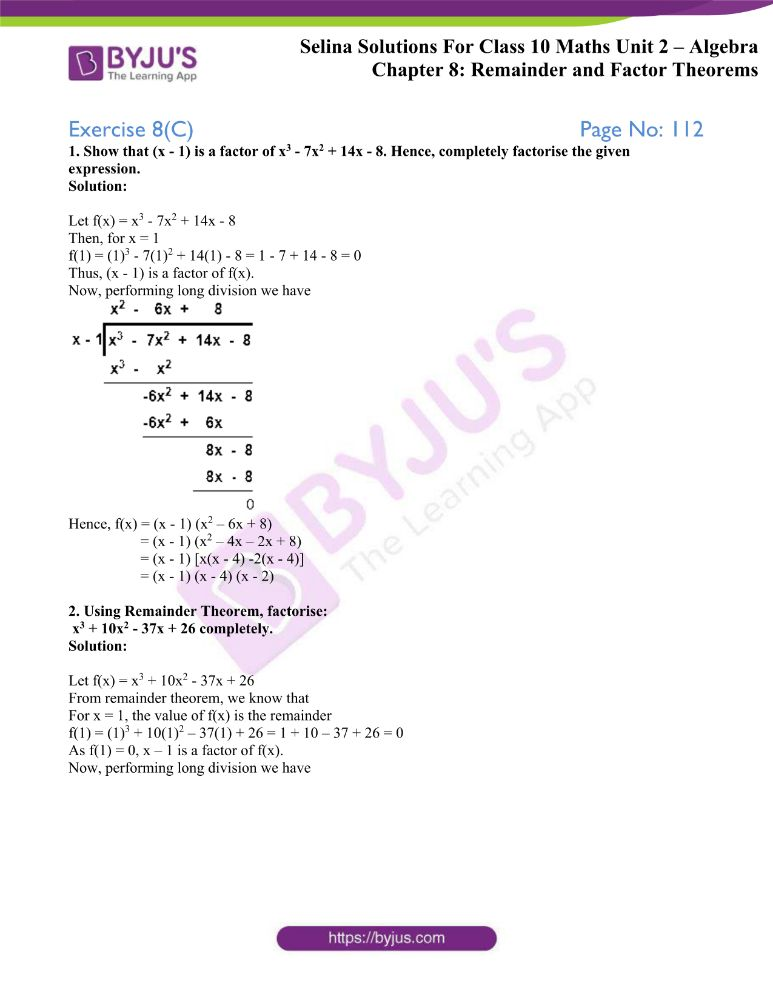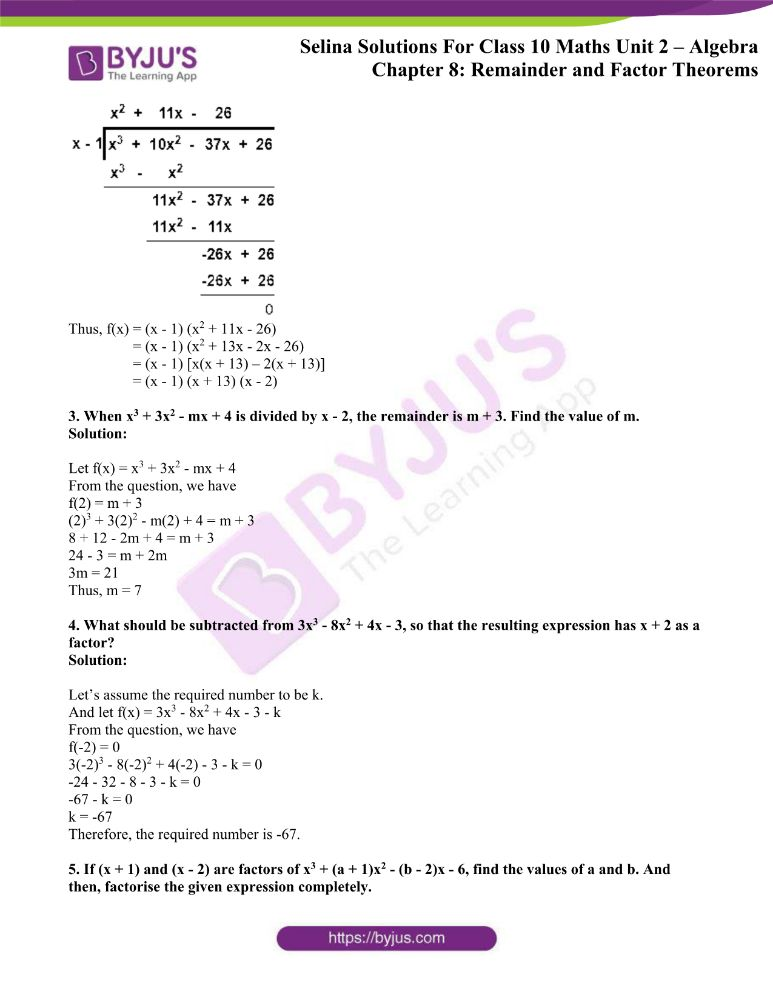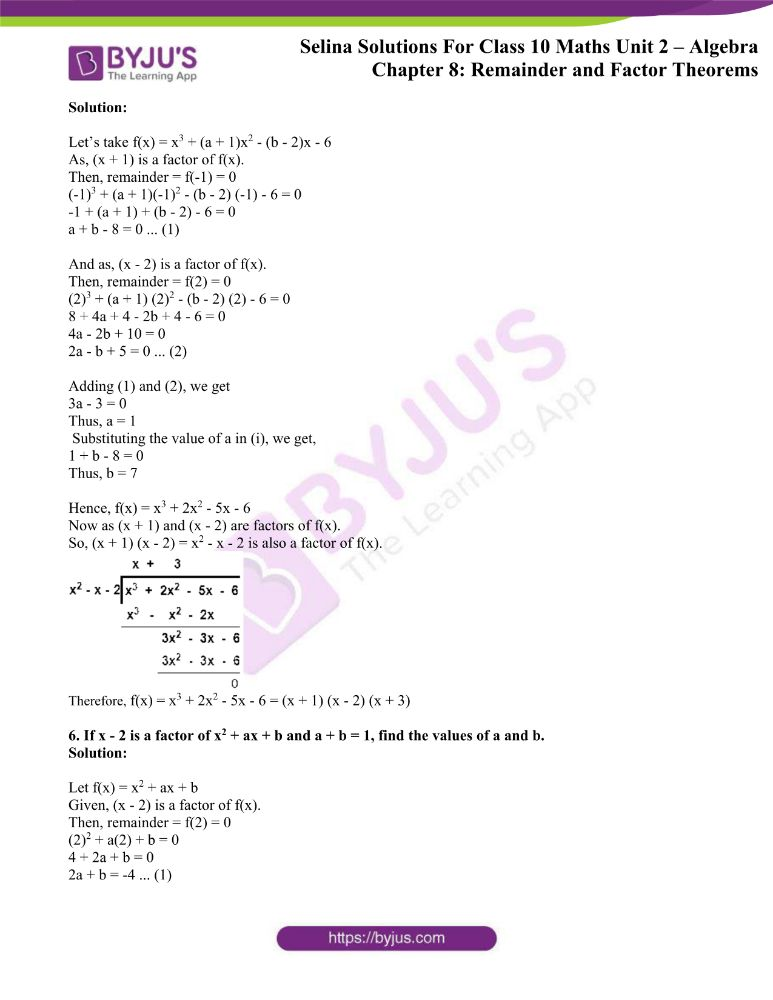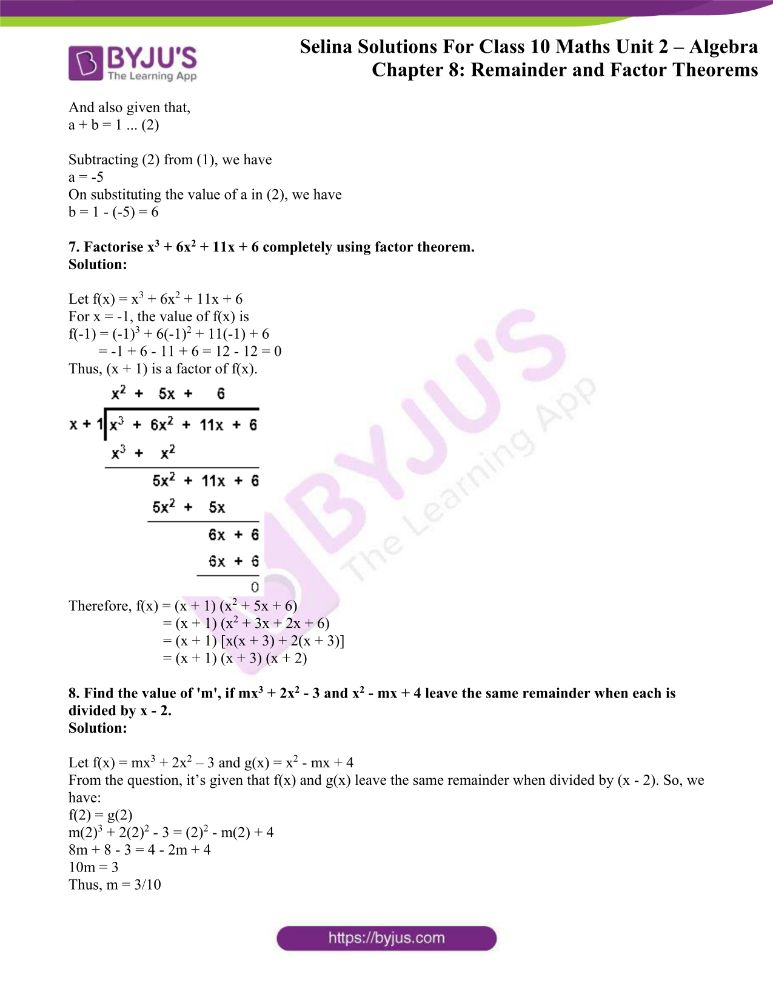### Exercises of Concise Selina Solutions Class 10 Maths Chapter 8 Remainder and Factor Theorems

Exercise 8(A) Solutions

Exercise 8(B) Solutions

Exercise 8(C) Solutions

## Access Selina Solutions Concise Maths Class 10 Chapter 8 Remainder and Factor Theorems

Exercise 8(A) Page No: 108

1. Find, in each case, the remainder when:

(i) x4 – 3x2 + 2x + 1 is divided by x – 1.

(ii) x3 + 3x2 – 12x + 4 is divided by x – 2.

(ii) x4 + 1 is divided by x + 1.

Solution:

From remainder theorem, we know that when a polynomial f (x) is divided by (x – a), then the remainder is f(a).

(i) Given, f(x) = x4 – 3x2 + 2x + 1 is divided by x – 1

So, remainder = f(1) = (1)4 – 3(1)2 + 2(1) + 1 = 1 – 3 + 2 + 1 = 1

(ii) Given, f(x) = x3 + 3x2 – 12x + 4 is divided by x – 2

So, remainder = f(2) = (2)3 + 3(2)2 – 12(2) + 4 = 8 + 12 – 24 + 4 = 0

(iii) Given, f(x) = x4 + 1 is divided by x + 1

So, remainder = f(-1) = (-1)4 + 1 = 2

2. Show that:

(i) x – 2 is a factor of 5x2 + 15x – 50

(ii) 3x + 2 is a factor of 3x2 – x – 2

Solution:

(x – a) is a factor of a polynomial f(x) if the remainder, when f(x) is divided by (x – a), is 0, i.e., if f(a) = 0.

(i) f(x) = 5x2 + 15x – 50

f(2) = 5(2)2 + 15(2) – 50 = 20 + 30 – 50 = 0

As the remainder is zero for x = 2

Thus, we can conclude that (x – 2) is a factor of 5x2 + 15x – 50

(ii) f(x) = 3x2 – x – 2

f(-2/3) = 3(-2/3)2 – (-2/3) – 2 = 4/3 + 2/3 – 2 = 2 – 2 = 0

As the remainder is zero for x = -2/3

Thus, we can conclude that (3x + 2) is a factor of 3x2 – x – 2

3. Use the Remainder Theorem to find which of the following is a factor of 2x3 + 3x2 – 5x – 6.

(i) x + 1 (ii) 2x – 1

(iii) x + 2

Solution:

From remainder theorem we know that when a polynomial f (x) is divided by x – a, then the remainder is f(a).

Here, f(x) = 2x3 + 3x2 – 5x – 6

(i) f (-1) = 2(-1)3 + 3(-1)2 – 5(-1) – 6 = -2 + 3 + 5 – 6 = 0

⇒ Remainder is zero for x = -1

Therefore, (x + 1) is a factor of the polynomial f(x).

(ii) f(1/2) = 2(1/2)3 + 3(1/2)2 – 5(1/2) – 6

= ¼ + ¾ – 5/2 – 6

= -5/2 – 5 = -15/2

⇒ Remainder is not equals to zero for x = 1/2

Therefore, (2x – 1) is not a factor of the polynomial f(x).

(iii) f (-2) = 2(-2)3 + 3(-2)2 – 5(-2) – 6 = -16 + 12 + 10 – 6 = 0

⇒ Remainder is zero for x = -2

Therefore, (x + 2) is a factor of the polynomial f(x).

4. (i) If 2x + 1 is a factor of 2x2 + ax – 3, find the value of a.

(ii) Find the value of k, if 3x – 4 is a factor of expression 3x2 + 2x – k.

Solution:

(i) Given, 2x + 1 is a factor of f(x) = 2x2 + ax – 3.

So, f(-1/2) = 0

2(-1/2)2 + a(-1/2) – 3 = 0

½ – a/2 – 3 = 0

1 – a – 6 = 0

a = -5

(ii) Given, 3x – 4 is a factor of g(x) = 3x2 + 2x – k.

So, f(4/3) = 0

3(4/3)2 + 2(4/3) – k = 0

16/3 + 8/3 – k = 0

24/3 = k

k = 8

5. Find the values of constants a and b when x – 2 and x + 3 both are the factors of expression x3 + ax2 + bx – 12.

Solution:

Here, f(x) = x3 + ax2 + bx – 12

Given, x – 2 and x + 3 both are the factors of f(x)

So,

f(2) and f(-3) both should be equal to zero.

f(2) = (2)3 + a(2)2 + b(2) – 12

0 = 8 + 4a + 2b – 12

0 = 4a + 2b – 4

2a + b = 2 …. (1)

Now,

f(-3) = (-3)3 + a(-3)2 + b(-3) – 12

0 = -27 + 9a – 3b – 12

9a – 3b – 39 = 0

3a – b = 13 …. (2)

Adding (1) and (2), we get,

5a = 15

Thus, a = 3

Putting the value of a in (1), we have

6 + b = 2

Thus, b = -4

6. Find the value of k, if 2x + 1 is a factor of (3k + 2)x3 + (k – 1).

Solution:

Let take f(x) = (3k + 2)x3 + (k – 1)

Now, 2x + 1 = 0

x = -1/2

As, 2x + 1 is a factor of f(x) then the remainder should be 0.

f(-1/2) = (3k + 2)(-1/2)3 + (k – 1) = 05k = 10 = 0

k = 2

7. Find the value of a, if x – 2 is a factor of 2x5 – 6x4 – 2ax3 + 6ax2 + 4ax + 8.

Solution:

Given, f(x) = 2x5 – 6x4 – 2ax3 + 6ax2 + 4ax + 8 and x – 2 is a factor of f(x).

So, x – 2 = 0; x = 2

Hence, f(2) = 0

2(2)5 – 6(2)4 – 2a(2)3 + 6a(2)2 + 4a(2) + 8 = 0

64 – 96 – 16a + 24a + 8a + 8 = 0

-24 + 16a = 0

16a = 24

Thus, a = 1.5

8. Find the values of m and n so that x – 1 and x + 2 both are factors of x3 + (3m + 1) x2 + nx – 18.

Solution:

Let f(x) = x3 + (3m + 1) x2 + nx – 18

Given, (x – 1) and (x + 2) are the factors of f(x).

So,

x – 1 = 0; x = 1 and x + 2 = 0; x = -2

f(1) and f(-2) both should be equal to zero.

(1)3 + (3m + 1)(1)2 + n(1) – 18 = 0

1 + 3m + 1 + n – 18 = 0

3m + n – 16 = 0 ….. (1)

And,

(-2)3 + (3m + 1)(-2)2 + n(-2) – 18 = 0

8 + 12m + 4 – 2n – 18 = 0

12m – 2n – 22 = 0

6m – n – 11 = 0 ….. (2)

Adding (1) and (2), we get,

9m – 27 = 0

Thus, m = 3

Putting the value of m in (1), we have

3(3) + n – 16 =0

9 + n – 16 = 0

Therefore, n = 7

Exercise 8(B) Page No: 111

1. Using the Factor Theorem, show that:

(i) (x – 2) is a factor of x3 – 2x2 – 9x + 18. Hence, factorise the expression x3 – 2x2 – 9x + 18 completely.

(ii) (x + 5) is a factor of 2x3 + 5x2 – 28x – 15. Hence, factorise the expression 2x3 + 5x2 – 28x – 15 completely.

(iii) (3x + 2) is a factor of 3x3 + 2x2 – 3x – 2. Hence, factorise the expression 3x3 + 2x2 – 3x – 2 completely.

Solution:

(i) Here, f(x) = x3 – 2x2 – 9x + 18

So, x – 2 = 0 ⇒ x = 2

Thus, remainder = f(2)

= (2)3 – 2(2)2 – 9(2) + 18

= 8 – 8 – 18 + 18

= 0

Therefore, (x – 2) is a factor of f(x).

Now, performing division of polynomial f(x) by (x – 2) we have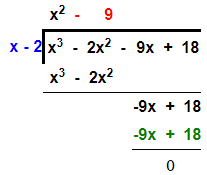Thus, x3 – 2x2 – 9x + 18 = (x – 2) (x2 – 9) = (x – 2) (x + 3) (x – 3)

(ii) Here, f(x) = 2x3 + 5x2 – 28x – 15

So, x + 5 = 0 ⇒ x = -5

Thus, remainder = f(-5)

= 2(-5)3 + 5(-5)2 – 28(-5) – 15

= -250 + 125 + 140 – 15

= -265 + 265

= 0

Therefore, (x + 5) is a factor of f(x).

Now, performing division of polynomial f(x) by (x + 5) we get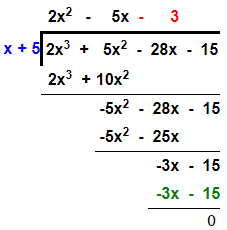So, 2x3 + 5x2 – 28x – 15 = (x + 5) (2x2 – 5x – 3)

Further, on factorisation

= (x + 5) [2x2 – 6x + x – 3]

= (x + 5) [2x(x – 3) + 1(x – 3)] = (x + 5) (2x + 1) (x – 3)

Thus, f(x) is factorised as (x + 5) (2x + 1) (x – 3)

(iii) Here, f(x) = 3x3 + 2x2 – 3x – 2

So, 3x + 2 = 0 ⇒ x = -2/3

Thus, remainder = f(-2/3)

= 3(-2/3)3 + 2(-2/3)2 – 3(-2/3) – 2

= -8/9 + 8/9 + 2 – 2

= 0

Therefore, (3x + 2) is a factor of f(x).

Now, performing division of polynomial f(x) by (3x + 2) we get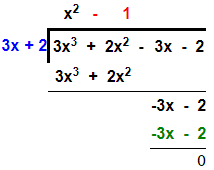Thus, 3x3 + 2x2 – 3x – 2 = (3x + 2) (x2 – 1) = (3x + 2) (x – 1) (x + 1)

2. Using the Remainder Theorem, factorise each of the following completely.

(i) 3x+ 2x2 − 19x + 6

(ii) 2x3 + x2 – 13x + 6

(iii) 3x3 + 2x2 – 23x – 30

(iv) 4x3 + 7x2 – 36x – 63

(v) x3 + x2 – 4x – 4

Solution:

(i) Let f(x) = 3x+ 2x2 − 19x + 6

For x = 2, the value of f(x) will be

= 3(2)+ 2(2)2 – 19(2) + 6

= 24 + 8 – 38 + 6 = 0

As f(2) = 0, so (x – 2) is a factor of f(x).

Now, performing long division we have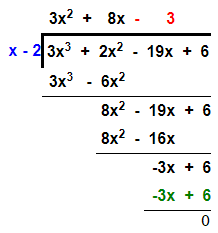Thus, f(x) = (x -2) (3x2 + 8x – 3)

= (x – 2) (3x2 + 9x – x – 3)

= (x – 2) [3x(x + 3) -1(x + 3)]

= (x – 2) (x + 3) (3x – 1)

(ii) Let f(x) = 2x3 + x2 – 13x + 6

For x = 2, the value of f(x) will be

f(2) = 2(2)3 + (2)2 – 13(2) + 6 = 16 + 4 – 26 + 6 = 0

As f(2) = 0, so (x – 2) is a factor of f(x).

Now, performing long division we have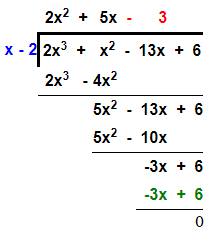Thus, f(x) = (x -2) (2x2 + 5x – 3)

= (x – 2) [2x2 + 6x – x – 3]

= (x – 2) [2x(x + 3) -1(x + 3)]

= (x – 2) [2x(x + 3) -1(x + 3)]

= (x – 2) (2x – 1) (x + 3)

(iii) Let f(x) = 3x3 + 2x2 – 23x – 30

For x = -2, the value of f(x) will be

f(-2) = 3(-2)3 + 2(-2)2 – 23(-2) – 30

= -24 + 8 + 46 – 30 = -54 + 54 = 0

As f(-2) = 0, so (x + 2) is a factor of f(x).

Now, performing long division we have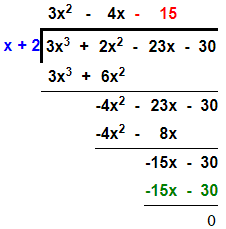Thus, f(x) = (x + 2) (3x2 – 4x – 15)

= (x + 2) (3x2 – 9x + 5x – 15)

= (x + 2) [3x(x – 3) + 5(x – 3)]

= (x + 2) (3x + 5) (x – 3)

(iv) Let f(x) = 4x3 + 7x2 – 36x – 63

For x = 3, the value of f(x) will be

f(3) = 4(3)3 + 7(3)2 – 36(3) – 63

= 108 + 63 – 108 – 63 = 0

As f(3) = 0, (x + 3) is a factor of f(x).

Now, performing long division we have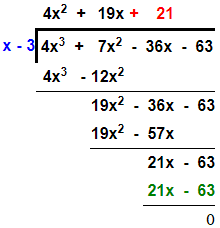Thus, f(x) = (x + 3) (4x2 – 5x – 21)

= (x + 3) (4x2 – 12x + 7x – 21)

= (x + 3) [4x(x – 3) + 7(x – 3)]

= (x + 3) (4x + 7) (x – 3)

(v) Let f(x) = x3 + x2 – 4x – 4

For x = -1, the value of f(x) will be

f(-1) = (-1)3 + (-1)2 – 4(-1) – 4

= -1 + 1 + 4 – 4 = 0

As, f(-1) = 0 so (x + 1) is a factor of f(x).

Now, performing long division we have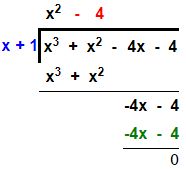Thus, f(x) = (x + 1) (x2 – 4)

= (x + 1) (x – 2) (x + 2)

3. Using the Remainder Theorem, factorise the expression 3x3 + 10x2 + x – 6. Hence, solve the equation 3x3 + 10x2 + x – 6 = 0.

Solution:

Let’s take f(x) = 3x3 + 10x2 + x – 6

For x = -1, the value of f(x) will be

f(-1) = 3(-1)3 + 10(-1)2 + (-1) – 6 = -3 + 10 – 1 – 6 = 0

As, f(-1) = 0 so (x + 1) is a factor of f(x).

Now, performing long division we have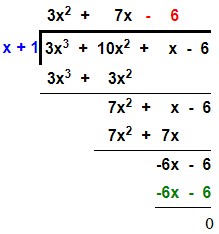Thus, f(x) = (x + 1) (3x2 + 7x – 6)

= (x + 1) (3x2 + 9x – 2x – 6)

= (x + 1) [3x(x + 3) -2(x + 3)]

= (x + 1) (x + 3) (3x – 2)

Now, 3x3 + 10x2 + x – 6 = 0

(x + 1) (x + 3) (3x – 2) = 0

Therefore,

x = -1, -3 or 2/3

4. Factorise the expression f (x) = 2x3 – 7x2 – 3x + 18. Hence, find all possible values of x for which f(x) = 0.

Solution:

Let f(x) = 2x3 – 7x2 – 3x + 18

For x = 2, the value of f(x) will be

f(2) = 2(2)3 – 7(2)2 – 3(2) + 18

= 16 – 28 – 6 + 18 = 0

As f(2) = 0, (x – 2) is a factor of f(x).

Now, performing long division we have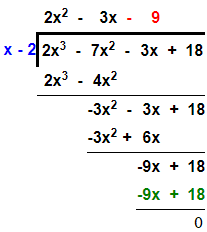Thus, f(x) = (x – 2) (2x2 – 3x – 9)

= (x – 2) (2x2 – 6x + 3x – 9)

= (x – 2) [2x(x – 3) + 3(x – 3)]

= (x – 2) (x – 3) (2x + 3)

Now, for f(x) = 0

(x – 2) (x – 3) (2x + 3) = 0

Hence x = 2, 3 or -3/2

5. Given that x – 2 and x + 1 are factors of f(x) = x3 + 3x2 + ax + b; calculate the values of a and b. Hence, find all the factors of f(x).

Solution:

Let f(x) = x3 + 3x2 + ax + b

As, (x – 2) is a factor of f(x), so f(2) = 0

(2)3 + 3(2)2 + a(2) + b = 0

8 + 12 + 2a + b = 0

2a + b + 20 = 0 … (1)

And as, (x + 1) is a factor of f(x), so f(-1) = 0

(-1)3 + 3(-1)2 + a(-1) + b = 0

-1 + 3 – a + b = 0

-a + b + 2 = 0 … (2)

Subtracting (2) from (1), we have

3a + 18 = 0

a = -6

On substituting the value of a in (ii), we have

b = a – 2 = -6 – 2 = -8

Thus, f(x) = x3 + 3x2 – 6x – 8

Now, for x = -1

f(-1) = (-1)3 + 3(-1)2 – 6(-1) – 8 = -1 + 3 + 6 – 8 = 0

Therefore, (x + 1) is a factor of f(x).

Now, performing long division we have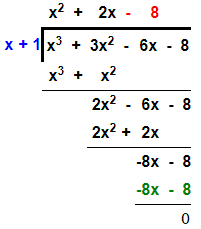Hence, f(x) = (x + 1) (x2 + 2x – 8)

= (x + 1) (x2 + 4x – 2x – 8)

= (x + 1) [x(x + 4) – 2(x + 4)]

= (x + 1) (x + 4) (x – 2)

Exercise 8(C) Page No: 112

1. Show that (x – 1) is a factor of x3 – 7x2 + 14x – 8. Hence, completely factorise the given expression.

Solution:

Let f(x) = x3 – 7x2 + 14x – 8

Then, for x = 1

f(1) = (1)3 – 7(1)2 + 14(1) – 8 = 1 – 7 + 14 – 8 = 0

Thus, (x – 1) is a factor of f(x).

Now, performing long division we have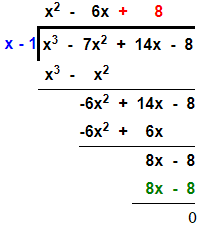Hence, f(x) = (x – 1) (x2 – 6x + 8)

= (x – 1) (x2 – 4x – 2x + 8)

= (x – 1) [x(x – 4) -2(x – 4)]

= (x – 1) (x – 4) (x – 2)

2. Using Remainder Theorem, factorise:

x3 + 10x2 – 37x + 26 completely.

Solution:

Let f(x) = x3 + 10x2 – 37x + 26

From remainder theorem, we know that

For x = 1, the value of f(x) is the remainder

f(1) = (1)3 + 10(1)2 – 37(1) + 26 = 1 + 10 – 37 + 26 = 0

As f(1) = 0, x – 1 is a factor of f(x).

Now, performing long division we have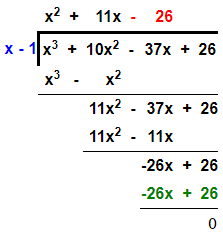Thus, f(x) = (x – 1) (x2 + 11x – 26)

= (x – 1) (x2 + 13x – 2x – 26)

= (x – 1) [x(x + 13) – 2(x + 13)]

= (x – 1) (x + 13) (x – 2)

3. When x3 + 3x2 – mx + 4 is divided by x – 2, the remainder is m + 3. Find the value of m.

Solution:

Let f(x) = x3 + 3x2 – mx + 4

From the question, we have

f(2) = m + 3

(2)3 + 3(2)2 – m(2) + 4 = m + 3

8 + 12 – 2m + 4 = m + 3

24 – 3 = m + 2m

3m = 21

Thus, m = 7

4. What should be subtracted from 3x3 – 8x2 + 4x – 3, so that the resulting expression has x + 2 as a factor?

Solution:

Let’s assume the required number to be k.

And let f(x) = 3x3 – 8x2 + 4x – 3 – k

From the question, we have

f(-2) = 0

3(-2)3 – 8(-2)2 + 4(-2) – 3 – k = 0

-24 – 32 – 8 – 3 – k = 0

-67 – k = 0

k = -67

Therefore, the required number is -67.

5. If (x + 1) and (x – 2) are factors of x3 + (a + 1)x2 – (b – 2)x – 6, find the values of a and b. And then, factorise the given expression completely.

Solution:

Let’s take f(x) = x3 + (a + 1)x2 – (b – 2)x – 6

As, (x + 1) is a factor of f(x).

Then, remainder = f(-1) = 0

(-1)3 + (a + 1)(-1)2 – (b – 2) (-1) – 6 = 0

-1 + (a + 1) + (b – 2) – 6 = 0

a + b – 8 = 0 … (1)

And as, (x – 2) is a factor of f(x).

Then, remainder = f(2) = 0

(2)3 + (a + 1) (2)2 – (b – 2) (2) – 6 = 0

8 + 4a + 4 – 2b + 4 – 6 = 0

4a – 2b + 10 = 0

2a – b + 5 = 0 … (2)

Adding (1) and (2), we get

3a – 3 = 0

Thus, a = 1

Substituting the value of a in (i), we get,

1 + b – 8 = 0

Thus, b = 7

Hence, f(x) = x3 + 2x2 – 5x – 6

Now as (x + 1) and (x – 2) are factors of f(x).

So, (x + 1) (x – 2) = x2 – x – 2 is also a factor of f(x).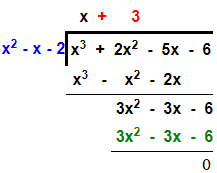Therefore, f(x) = x3 + 2x2 – 5x – 6 = (x + 1) (x – 2) (x + 3)

6. If x – 2 is a factor of x2 + ax + b and a + b = 1, find the values of a and b.

Solution:

Let f(x) = x2 + ax + b

Given, (x – 2) is a factor of f(x).

Then, remainder = f(2) = 0

(2)2 + a(2) + b = 0

4 + 2a + b = 0

2a + b = -4 … (1)

And also given that,

a + b = 1 … (2)

Subtracting (2) from (1), we have

a = -5

On substituting the value of a in (2), we have

b = 1 – (-5) = 6

7. Factorise x3 + 6x2 + 11x + 6 completely using factor theorem.

Solution:

Let f(x) = x3 + 6x2 + 11x + 6

For x = -1, the value of f(x) is

f(-1) = (-1)3 + 6(-1)2 + 11(-1) + 6

= -1 + 6 – 11 + 6 = 12 – 12 = 0

Thus, (x + 1) is a factor of f(x).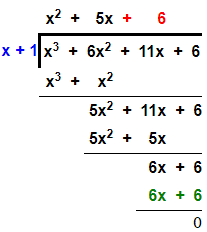Therefore, f(x) = (x + 1) (x2 + 5x + 6)

= (x + 1) (x2 + 3x + 2x + 6)

= (x + 1) [x(x + 3) + 2(x + 3)]

= (x + 1) (x + 3) (x + 2)

8. Find the value of ‘m’, if mx3 + 2x2 – 3 and x2 – mx + 4 leave the same remainder when each is divided by x – 2.

Solution:

Let f(x) = mx3 + 2x2 – 3 and g(x) = x2 – mx + 4

From the question, it’s given that f(x) and g(x) leave the same remainder when divided by (x – 2). So, we have:

f(2) = g(2)

m(2)3 + 2(2)2 – 3 = (2)2 – m(2) + 4

8m + 8 – 3 = 4 – 2m + 4

10m = 3

Thus, m = 3/10

The given solutions are as per the 2019-20 Concise Selina textbook. The Selina Solutions for the academic year 2020-21 will be updated soon.